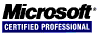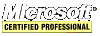# .NET C# Guide

### Inheritance

Inheritance is one of the important principles of OOP. The class being inherited is called the base class and the class that is doing the inheriting is called the derived class. To have a new `Box` class inherit the `Rectangle` class follow this code:

``````class Rectangle
{
private int width, height;
protected string name; // CHANGE THIS TO A protected MEMBER

public Rectangle()
{
Width = Height = 1;
}

public Rectangle(int x, int y)
{
Width = x;
Height = y;
}

~Rectangle()
{
// do something
}

// change this into a property to be consistent
public int Area
{
get
{
return Width * Height;
}
}

// Width property
public int Width
{
get
{
return width;
}
set
{
if (value > 0)
width = value;
}
}

// Height property
public int Height
{
get
{
return height;
}
set
{
if (value > 0)
height = value;
}
}
}

// Box is the derived class and Rectangle is the base class
class Box : Rectangle
{
private int depth;

public Box() : base()
{
name = "box";
Depth = 1;
}

public Box(int x, int y, int z) : base(x, y)
{
name = "box";
Depth = z;
}

public int Depth
{
get
{
return depth;
}
set
{
if (value > 0)
depth = value;
}
}

new public int Area
{
get
{
return (Width * Height) * 2 + (Width * Depth) * 2 + (Height * Depth) * 2;
}
}

public int Volume
{
get
{
// return the volume of the box
return Width * Height * Depth;
}
}
}
``````

There are a few new keywords to go over. The `protected` access modifier lets the derived class have access to the data member which is not available outside the derived class or the base class. The `new` keyword allows the derived class to define a method or property with the same so that the `Area` property returns the area of the box and not the rectangle. The `base` keyword is used here to access the parameterized constructor of the base class.

#### Example Program Code

Run this program to see when constructors and destructors are called when inheritance is involved.

``````using System;

namespace ConsoleApplication1
{
class Rectangle
{
private int width, height;
protected string name; // CHANGE THIS TO A protected MEMBER

public Rectangle()
{
Width = Height = 1;
Console.WriteLine("Rectangle()");
}

public Rectangle(int x, int y)
{
Width = x;
Height = y;
Console.WriteLine("Rectangle(int x, int y)");
}

~Rectangle()
{
Console.WriteLine("~Rectangle()");
}

// change this into a property to be consistent
public int Area
{
get
{
return Width * Height;
}
}

// Width property
public int Width
{
get
{
return width;
}
set
{
if (value > 0)
width = value;
}
}

// Height property
public int Height
{
get
{
return height;
}
set
{
if (value > 0)
height = value;
}
}
}

// Box is the derived class and Rectangle is the base class
class Box : Rectangle
{
private int depth;

public Box() : base()
{
Console.WriteLine("Box()");
name = "box";
Depth = 1;
}

public Box(int x, int y, int z) : base(x, y)
{
Console.WriteLine("Box(int x, int y, int z)");
name = "box";
Depth = z;
}

public int Depth
{
get
{
return depth;
}
set
{
if (value > 0)
depth = value;
}
}

new public int Area
{
get
{
return (Width * Height) * 2 + (Width * Depth) * 2 + (Height * Depth) * 2;
}
}

public int Volume
{
get
{
// return the volume of the box
return Width * Height * Depth;
}
}
}

class Program
{
static void Main(string[] args)
{
Box box1 = new ConsoleApplication1.Box();
Box box2 = new ConsoleApplication1.Box(10, 5, 20);

Console.WriteLine("box1 Area: " + box1.Area);
Console.WriteLine("box1 Volume: " + box1.Volume);

Console.WriteLine("box2 Area: " + box2.Area);
Console.WriteLine("box2 Volume: " + box2.Volume);
}
}
}
``````#### Virtual Methods and Overriding

A virtual method is one that is declared using the `virtual` keyword in a base class and redefined, via the `override` keyword, in one or more derived classes. This means that each derived class can have its own version of a virtual method. This is how C# implements polymorphism. An example of it follows.

#### Example Program Code

``````using System;

namespace ConsoleApplication1
{
class Base
{
virtual public void Name()
{
Console.WriteLine("My Name is Base");
}
}

class A : Base
{
public override void Name()
{
Console.WriteLine("My Name is A");
}
}

class B : Base
{
public override void Name()
{
Console.WriteLine("My Name is B");
}
}

class C : Base
{
public override void Name()
{
Console.WriteLine("My Name is C");
}
}

class Program
{
static void Main(string[] args)
{
Base o = new Base();
A a = new A();
B b = new B();
C c = new C();

Base reference;

reference = o;
reference.Name();

reference = a;
reference.Name();

reference = b;
reference.Name();

reference = c;
reference.Name();
}
}
}
``````#### Abstract Classes

Abstract classes are base classes which require the derived classes to implement its methods.

``````abstract class Base
{
public abstract void Name();
}

class Derived : Base
{
// if the abstract method Name() is not
// overridden then it will not compile
public override void Name()
{
Console.WriteLine("My Name is Derived");
}
}
``````

#### Preventing Inheritance

Use the keyword `sealed` to prevent other classes from inheriting a class.

``````sealed class A
{
public void Name()
{
Console.WriteLine("My Name is A");
}
}

class B : A
{
// this will not compile because A is "sealed"
}
``````
<< < [Page 6 of 7] > >>